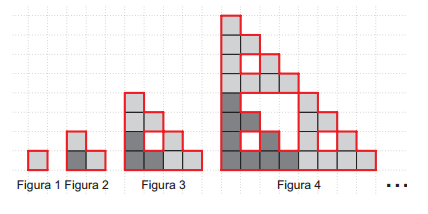# 8- A Pattern

Geometry Level 2In the pattern above, each figure after the second is formed by joining three copies of the previous.

The perimeter of each figure is marked in red. The perimeter of the 1st figure is 4, of the 2nd is 8, of the 3rd is 20 and of the 4th is 56.

What is the perimeter of the 6th figure?

×